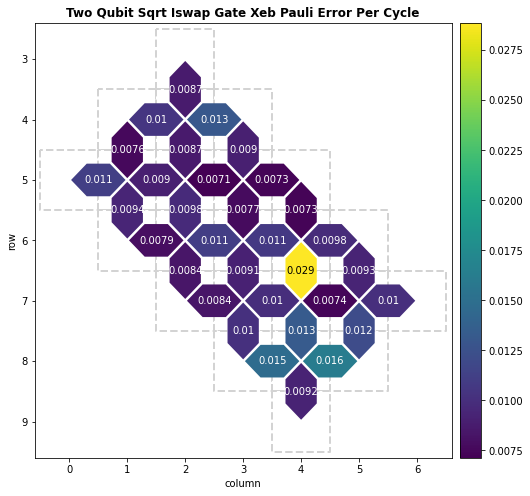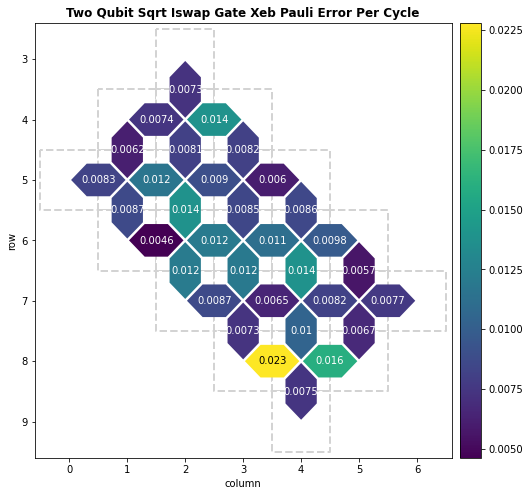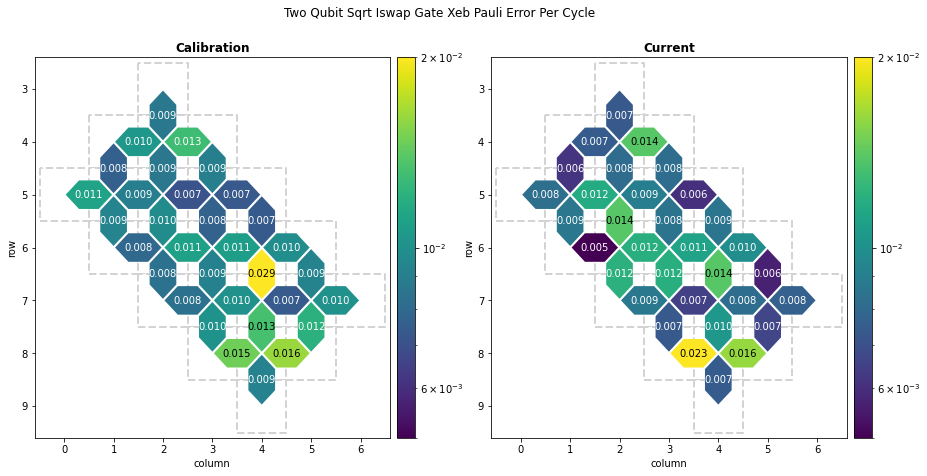Comparing current processor performance to the latest calibration data

Calibration runs at periodic intervals, but processor performances changes over time. How does the current state of a processor compare to the latest calibration data? This tutorial shows how to run parallel XEB and compare current two-qubit gate errors to the most recent calibration report.

Disclaimer: The data shown in this tutorial is exemplary and not representative of the QCS in production.

Setup

First, we install Cirq and import the packages we will use.

try:
import cirq
except ImportError:
!pip install --quiet cirq --pre
import matplotlib.pyplot as plt
import networkx as nx
import numpy as np

import cirq

Next, we authorize to use the Quantum Computing Service.

Getting OAuth2 credentials.
Press enter after entering the verification code.
Authentication complete.

Now, we select a processor and visualize a two-qubit gate calibration metric. See the Visualizing calibration metrics tutorial for more background.Parallel XEB

After viewing the latest calibration data, we now run parallel XEB on a set of qubits on the processor. Note that you can select a subset of qubits on the device which will shorten the runtime of the experiment.

"""Select qubits to run parallel XEB on."""
qubits = cg.get_engine_device(processor_id).qubit_set()
"""Setup for parallel XEB experiment."""
from cirq.experiments import random_quantum_circuit_generation as rqcg
import cirq.contrib.routing as ccr

# Generate library of XEB circuits.
circuit_library = rqcg.generate_library_of_2q_circuits(
n_library_circuits=20,
two_qubit_gate=cirq.SQRT_ISWAP,
random_state=52,
)
combs_by_layer = rqcg.get_random_combinations_for_device(
n_library_circuits=len(circuit_library),
n_combinations=10,
device_graph=ccr.gridqubits_to_graph_device(sorted(qubits)),
random_state=53,
)
cycle_depths = np.arange(3, 100, 20)
"""Collect all data by executing circuits."""
from cirq.experiments.xeb_sampling import sample_2q_xeb_circuits
from cirq.experiments.xeb_fitting import benchmark_2q_xeb_fidelities, fit_exponential_decays

sampler = cg.get_engine_sampler(
project_id=project_id,
processor_id=processor_id,
gate_set_name="sqrt_iswap",
)

# Run XEB circuits on the processor.
sampled_df = sample_2q_xeb_circuits(
sampler=sampler,
circuits=circuit_library,
cycle_depths=cycle_depths,
combinations_by_layer=combs_by_layer,
shuffle=np.random.RandomState(52),
)

# Run XEB circuits on a simulator and fit exponential decays.
fids = benchmark_2q_xeb_fidelities(
sampled_df=sampled_df,
circuits=circuit_library,
cycle_depths=cycle_depths,
)
fidelities = fit_exponential_decays(fids)
100%|██████████| 207/207 [22:55<00:00,  6.65s/it]

We can visualize these results on a cirq.TwoQubitInteractionHeatmap as follows.

"""Grab (pair, sqrt_iswap_pauli_error_per_cycle) data for all qubit pairs."""
pxeb_results = {
pair: (1.0 - fidelity) / (4 / 3)
for (_, _, pair), fidelity in fidelities.layer_fid.items()
}

cirq.TwoQubitInteractionHeatmap(pxeb_results).plot(
title=metric.replace("_", " ").title()
);Side by side comparison

To make it easy to compare, we plot both heatmaps side by side on the same color scale.

from matplotlib.colors import LogNorm

# Plot options.
vmin = 5e-3
vmax = 2e-2
options = {"norm": LogNorm()}
format = "0.3f"

fig, axes = plt.subplots(nrows=1, ncols=2, figsize=(15, 7))
fig.suptitle(metric.replace("_", " ").title())

calibration.heatmap(metric).plot(
ax=axes, title="Calibration", vmin=vmin, vmax=vmax,
collection_options=options, annotation_format=format,
)
cirq.TwoQubitInteractionHeatmap(pxeb_results).plot(
ax=axes, title="Current", vmin=vmin, vmax=vmax,
collection_options=options, annotation_format=format,
);We can now inspect these heatmaps to directly compare current processor performance to the latest calibration data.

[{ "type": "thumb-down", "id": "missingTheInformationINeed", "label":"Missing the information I need" },{ "type": "thumb-down", "id": "tooComplicatedTooManySteps", "label":"Too complicated / too many steps" },{ "type": "thumb-down", "id": "outOfDate", "label":"Out of date" },{ "type": "thumb-down", "id": "samplesCodeIssue", "label":"Samples / code issue" },{ "type": "thumb-down", "id": "otherDown", "label":"Other" }]
[{ "type": "thumb-up", "id": "easyToUnderstand", "label":"Easy to understand" },{ "type": "thumb-up", "id": "solvedMyProblem", "label":"Solved my problem" },{ "type": "thumb-up", "id": "otherUp", "label":"Other" }]